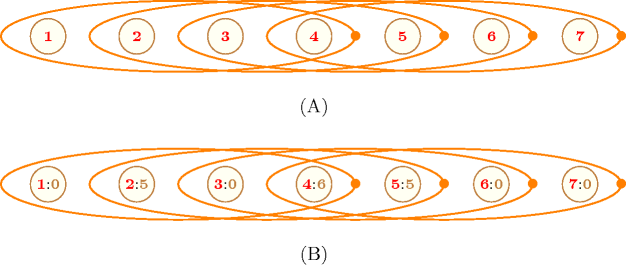## 5.350. sliding_distribution

Origin
Constraint

$\mathrm{𝚜𝚕𝚒𝚍𝚒𝚗𝚐}_\mathrm{𝚍𝚒𝚜𝚝𝚛𝚒𝚋𝚞𝚝𝚒𝚘𝚗}\left(\mathrm{𝚂𝙴𝚀},\mathrm{𝚅𝙰𝚁𝙸𝙰𝙱𝙻𝙴𝚂},\mathrm{𝚅𝙰𝙻𝚄𝙴𝚂}\right)$

Arguments
 $\mathrm{𝚂𝙴𝚀}$ $\mathrm{𝚒𝚗𝚝}$ $\mathrm{𝚅𝙰𝚁𝙸𝙰𝙱𝙻𝙴𝚂}$ $\mathrm{𝚌𝚘𝚕𝚕𝚎𝚌𝚝𝚒𝚘𝚗}\left(\mathrm{𝚟𝚊𝚛}-\mathrm{𝚍𝚟𝚊𝚛}\right)$ $\mathrm{𝚅𝙰𝙻𝚄𝙴𝚂}$ $\mathrm{𝚌𝚘𝚕𝚕𝚎𝚌𝚝𝚒𝚘𝚗}\left(\mathrm{𝚟𝚊𝚕}-\mathrm{𝚒𝚗𝚝},\mathrm{𝚘𝚖𝚒𝚗}-\mathrm{𝚒𝚗𝚝},\mathrm{𝚘𝚖𝚊𝚡}-\mathrm{𝚒𝚗𝚝}\right)$
Restrictions
 $\mathrm{𝚂𝙴𝚀}>0$ $\mathrm{𝚂𝙴𝚀}\le |\mathrm{𝚅𝙰𝚁𝙸𝙰𝙱𝙻𝙴𝚂}|$ $\mathrm{𝚛𝚎𝚚𝚞𝚒𝚛𝚎𝚍}$$\left(\mathrm{𝚅𝙰𝚁𝙸𝙰𝙱𝙻𝙴𝚂},\mathrm{𝚟𝚊𝚛}\right)$ $|\mathrm{𝚅𝙰𝙻𝚄𝙴𝚂}|>0$ $\mathrm{𝚛𝚎𝚚𝚞𝚒𝚛𝚎𝚍}$$\left(\mathrm{𝚅𝙰𝙻𝚄𝙴𝚂},\left[\mathrm{𝚟𝚊𝚕},\mathrm{𝚘𝚖𝚒𝚗},\mathrm{𝚘𝚖𝚊𝚡}\right]\right)$ $\mathrm{𝚍𝚒𝚜𝚝𝚒𝚗𝚌𝚝}$$\left(\mathrm{𝚅𝙰𝙻𝚄𝙴𝚂},\mathrm{𝚟𝚊𝚕}\right)$ $\mathrm{𝚅𝙰𝙻𝚄𝙴𝚂}.\mathrm{𝚘𝚖𝚒𝚗}\ge 0$ $\mathrm{𝚅𝙰𝙻𝚄𝙴𝚂}.\mathrm{𝚘𝚖𝚊𝚡}\le \mathrm{𝚂𝙴𝚀}$ $\mathrm{𝚅𝙰𝙻𝚄𝙴𝚂}.\mathrm{𝚘𝚖𝚒𝚗}\le \mathrm{𝚅𝙰𝙻𝚄𝙴𝚂}.\mathrm{𝚘𝚖𝚊𝚡}$
Purpose

For each sequence of $\mathrm{𝚂𝙴𝚀}$ consecutive variables of the $\mathrm{𝚅𝙰𝚁𝙸𝙰𝙱𝙻𝙴𝚂}$ collection, each value $\mathrm{𝚅𝙰𝙻𝚄𝙴𝚂}\left[i\right].\mathrm{𝚟𝚊𝚕}$ $\left(1\le i\le |\mathrm{𝚅𝙰𝙻𝚄𝙴𝚂}|\right)$ should be taken by at least $\mathrm{𝚅𝙰𝙻𝚄𝙴𝚂}\left[i\right].\mathrm{𝚘𝚖𝚒𝚗}$ and at most $\mathrm{𝚅𝙰𝙻𝚄𝙴𝚂}\left[i\right].\mathrm{𝚘𝚖𝚊𝚡}$ variables.

Example
$\left(\begin{array}{c}4,〈0,5,0,6,5,0,0〉,\hfill \\ 〈\begin{array}{ccc}\mathrm{𝚟𝚊𝚕}-0\hfill & \mathrm{𝚘𝚖𝚒𝚗}-1\hfill & \mathrm{𝚘𝚖𝚊𝚡}-2,\hfill \\ \mathrm{𝚟𝚊𝚕}-1\hfill & \mathrm{𝚘𝚖𝚒𝚗}-0\hfill & \mathrm{𝚘𝚖𝚊𝚡}-4,\hfill \\ \mathrm{𝚟𝚊𝚕}-4\hfill & \mathrm{𝚘𝚖𝚒𝚗}-0\hfill & \mathrm{𝚘𝚖𝚊𝚡}-4,\hfill \\ \mathrm{𝚟𝚊𝚕}-5\hfill & \mathrm{𝚘𝚖𝚒𝚗}-1\hfill & \mathrm{𝚘𝚖𝚊𝚡}-2,\hfill \\ \mathrm{𝚟𝚊𝚕}-6\hfill & \mathrm{𝚘𝚖𝚒𝚗}-0\hfill & \mathrm{𝚘𝚖𝚊𝚡}-2\hfill \end{array}〉\hfill \end{array}\right)$

The $\mathrm{𝚜𝚕𝚒𝚍𝚒𝚗𝚐}_\mathrm{𝚍𝚒𝚜𝚝𝚛𝚒𝚋𝚞𝚝𝚒𝚘𝚗}$ constraint holds since:

• On the first sequence of 4 consecutive values $0506$ values 0, 1, 4, 5 and 6 are respectively used 2, 0, 0, 1 and 1 times.

• On the second sequence of 4 consecutive values $5065$ values 0, 1, 4, 5 and 6 are respectively used 1, 0, 0, 2 and 1 times.

• On the third sequence of 4 consecutive values $0650$ values 0, 1, 4, 5 and 6 are respectively used 2, 0, 0, 1 and 1 times.

• On the fourth sequence of 4 consecutive values $6500$ values 0, 1, 4, 5 and 6 are respectively used 2, 0, 0, 1 and 1 times.

Typical
 $\mathrm{𝚂𝙴𝚀}>1$ $\mathrm{𝚂𝙴𝚀}<|\mathrm{𝚅𝙰𝚁𝙸𝙰𝙱𝙻𝙴𝚂}|$ $|\mathrm{𝚅𝙰𝚁𝙸𝙰𝙱𝙻𝙴𝚂}|>|\mathrm{𝚅𝙰𝙻𝚄𝙴𝚂}|$
Symmetries
• Items of $\mathrm{𝚅𝙰𝚁𝙸𝙰𝙱𝙻𝙴𝚂}$ can be reversed.

• An occurrence of a value of $\mathrm{𝚅𝙰𝚁𝙸𝙰𝙱𝙻𝙴𝚂}.\mathrm{𝚟𝚊𝚛}$ that does not belong to $\mathrm{𝚅𝙰𝙻𝚄𝙴𝚂}.\mathrm{𝚟𝚊𝚕}$ can be replaced by any other value that also does not belong to $\mathrm{𝚅𝙰𝙻𝚄𝙴𝚂}.\mathrm{𝚟𝚊𝚕}$.

• Items of $\mathrm{𝚅𝙰𝙻𝚄𝙴𝚂}$ are permutable.

• $\mathrm{𝚅𝙰𝙻𝚄𝙴𝚂}.\mathrm{𝚘𝚖𝚒𝚗}$ can be decreased to any value $\ge 0$.

• $\mathrm{𝚅𝙰𝙻𝚄𝙴𝚂}.\mathrm{𝚘𝚖𝚊𝚡}$ can be increased to any value $\le \mathrm{𝚂𝙴𝚀}$.

• All occurrences of two distinct values in $\mathrm{𝚅𝙰𝚁𝙸𝙰𝙱𝙻𝙴𝚂}.\mathrm{𝚟𝚊𝚛}$ or $\mathrm{𝚅𝙰𝙻𝚄𝙴𝚂}.\mathrm{𝚟𝚊𝚕}$ can be swapped; all occurrences of a value in $\mathrm{𝚅𝙰𝚁𝙸𝙰𝙱𝙻𝙴𝚂}.\mathrm{𝚟𝚊𝚛}$ or $\mathrm{𝚅𝙰𝙻𝚄𝙴𝚂}.\mathrm{𝚟𝚊𝚕}$ can be renamed to any unused value.

Arg. properties
• Contractible wrt. $\mathrm{𝚅𝙰𝚁𝙸𝙰𝙱𝙻𝙴𝚂}$ when $\mathrm{𝚂𝙴𝚀}=1$.

• Prefix-contractible wrt. $\mathrm{𝚅𝙰𝚁𝙸𝙰𝙱𝙻𝙴𝚂}$.

• Suffix-contractible wrt. $\mathrm{𝚅𝙰𝚁𝙸𝙰𝙱𝙻𝙴𝚂}$.

• Contractible wrt. $\mathrm{𝚅𝙰𝙻𝚄𝙴𝚂}$.

specialisation: $\mathrm{𝚊𝚖𝚘𝚗𝚐}_\mathrm{𝚜𝚎𝚚}$ (individual values replaced by single set of values).

Keywords
Arc input(s)

$\mathrm{𝚅𝙰𝚁𝙸𝙰𝙱𝙻𝙴𝚂}$

Arc generator
$\mathrm{𝑃𝐴𝑇𝐻}$$↦\mathrm{𝚌𝚘𝚕𝚕𝚎𝚌𝚝𝚒𝚘𝚗}$

Arc arity
$\mathrm{𝚂𝙴𝚀}$
Arc constraint(s)
$\mathrm{𝚐𝚕𝚘𝚋𝚊𝚕}_\mathrm{𝚌𝚊𝚛𝚍𝚒𝚗𝚊𝚕𝚒𝚝𝚢}_\mathrm{𝚕𝚘𝚠}_\mathrm{𝚞𝚙}$$\left(\mathrm{𝚌𝚘𝚕𝚕𝚎𝚌𝚝𝚒𝚘𝚗},\mathrm{𝚅𝙰𝙻𝚄𝙴𝚂}\right)$
Graph property(ies)
$\mathrm{𝐍𝐀𝐑𝐂}$$=|\mathrm{𝚅𝙰𝚁𝙸𝙰𝙱𝙻𝙴𝚂}|-\mathrm{𝚂𝙴𝚀}+1$

Graph model

Note that the $\mathrm{𝚜𝚕𝚒𝚍𝚒𝚗𝚐}_\mathrm{𝚍𝚒𝚜𝚝𝚛𝚒𝚋𝚞𝚝𝚒𝚘𝚗}$ constraint is a constraint where the arc constraints do not have an arity of 2.

Parts (A) and (B) of Figure 5.350.1 respectively show the initial and final graph associated with the Example slot. Since all arc constraints hold (i.e., because of the graph property $\mathrm{𝐍𝐀𝐑𝐂}=|\mathrm{𝚅𝙰𝚁𝙸𝙰𝙱𝙻𝙴𝚂}|-\mathrm{𝚂𝙴𝚀}+1$) the final graph corresponds to the initial graph.

##### Figure 5.350.1. (A) Initial and (B) final graph of the $\mathrm{𝚜𝚕𝚒𝚍𝚒𝚗𝚐}_\mathrm{𝚍𝚒𝚜𝚝𝚛𝚒𝚋𝚞𝚝𝚒𝚘𝚗}$$\left(\mathbf{4},〈0,\mathbf{5},\mathbf{0},\mathbf{6},\mathbf{5},\mathbf{0},\mathbf{0}〉,〈012,104,404,512,602〉\right)$ constraint of the Example slot where each ellipse represents an hyperedge involving $\mathrm{𝚂𝙴𝚀}=\mathbf{4}$ vertices (to each ellipse corresponds a $\mathrm{𝚐𝚕𝚘𝚋𝚊𝚕}_\mathrm{𝚌𝚊𝚛𝚍𝚒𝚗𝚊𝚕𝚒𝚝𝚢}_\mathrm{𝚕𝚘𝚠}_\mathrm{𝚞𝚙}$ constraint)